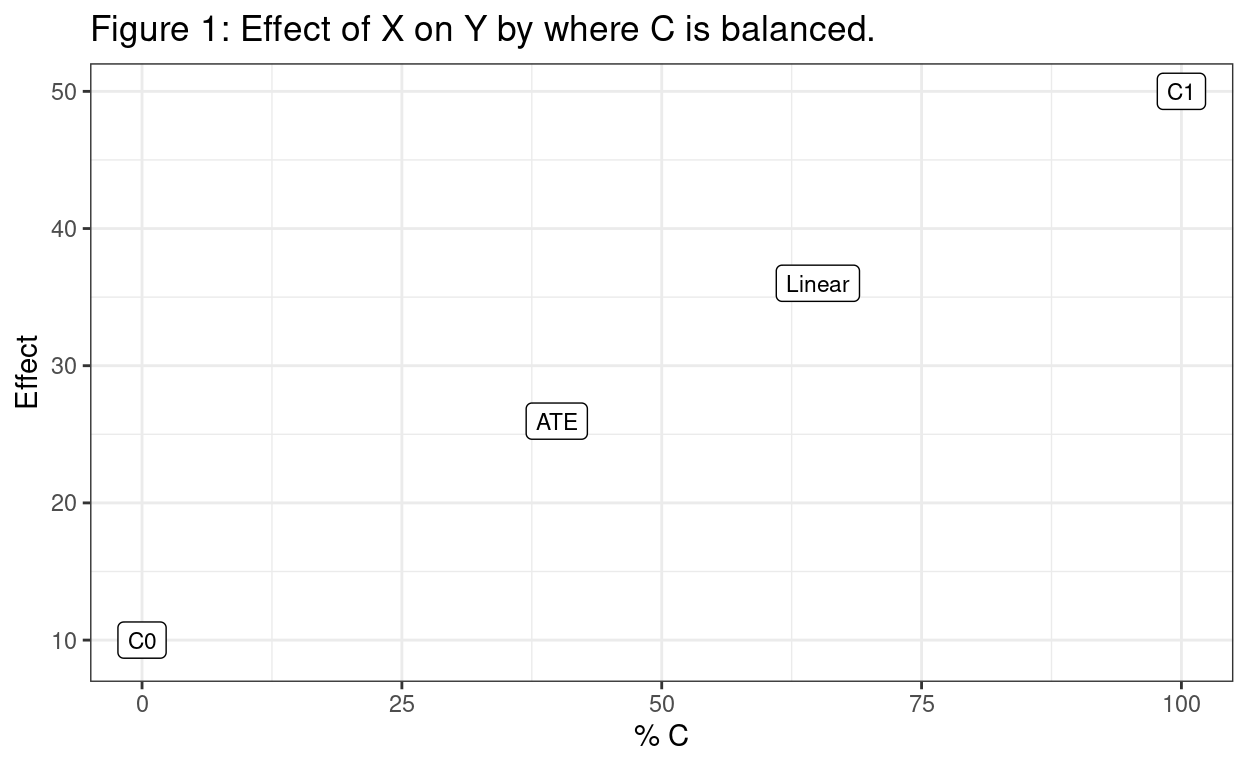# That’s not my population. Its variance is constant

Frank Popham
2021-04-24

Disclaimer: This is a blog and I am a quantitative social scientist not a statistician. All this means that there may be errors, my notation is probably wrong etc.

#### Not my population

Often epidemiologists study the effect of an exposure on an outcome in observational data using a regression model which also adjusts for confounders. But what population, in terms of the confounders, does this effect represent? In my simple example we want to know the effect of a binary exposure $$\operatorname{(X)}$$ on a continuous outcome $$\operatorname{(Y)}$$ with a binary confounder $$\operatorname{(C)}$$ using a linear regression.

$E( \operatorname{Y} ) = \alpha + \beta_{1}(\operatorname{X}) + \beta_{2}(\operatorname{C})$

As table 1 shows the percentage of $$\operatorname{C}$$ in the exposed (77%) is greater than in the unexposed (27%). We need to balance $$\operatorname{C}$$ over $$\operatorname{X}$$, and if we want the average treatment effect for the population then we need to balance at the average of $$\operatorname{C}$$ in the population. Our target population is $$\operatorname{C}$$ equals 40%. Although the linear regression does balance $$\operatorname{C}$$ it does so at a mean of 65%.

Table 1: Confounder balance before and after linear regression
Unexposed Exposed Unexposed Exposed
Confounder mean 27% 77% 40% 65% 65%

To work out where the linear regression is balancing I adapt this method. First, we run a linear regression of the confounder on the exposure.

$E( \operatorname{X} ) = \alpha + \beta_{1}(\operatorname{C})$

Second, we use the absolute value of the residual as a weight to find the weighted mean of $$\operatorname{C}$$ over $$\operatorname{X}$$.

#### Working residual

Effectively we are modelling a binary exposure using a linear regression with the assumption that residuals are constant over $$\operatorname{C}$$. However this is unlikely, and it may be better to use a logistic regression that does not assume a constant residual variance.

$\log\left[ \frac { P( \operatorname{X} = \operatorname{1} ) }{ 1 - P( \operatorname{X} = \operatorname{1} ) } \right] = \alpha + \beta_{1}(\operatorname{C})$

The working residual from the above is

$\frac { \operatorname{X} - \operatorname{\hat{X}} } { \operatorname{\hat{X}} * (1 - \operatorname{\hat{X}})}$

where $$\operatorname{\hat{X}}$$ is the prediction of $$\operatorname{X}$$. That is the residual divided by the variance of the prediction of $$\operatorname{\hat{X}}$$.

When $$\operatorname{X}$$ is 1 the working residual simplifies to $\frac{ \operatorname{1}}{\operatorname{\hat{X}}}$

which is the inverse probability weight and when $$\operatorname{X}$$ is 0 it simplifies to

$-\frac{ \operatorname{1}}{\operatorname{1} - \operatorname{\hat{X}}}$

which is the negative of the inverse probability weight. If we derive the weighted mean of $$\operatorname{C}$$ over $$\operatorname{X}$$ using the working residuals then we balance $$\operatorname{C}$$ and at the target population.

Table 2: Confounder balance before and after IPW
Unexposed Exposed Unexposed Exposed
Confounder mean 27% 77% 40% 40% 40%

#### Does it matter?

To obtain the effect of $$\operatorname{X}$$ on $$\operatorname{Y}$$ for our target population we can use the working residual as a weight in a linear regression of $$\operatorname{X}$$ on $$\operatorname{Y}$$ (i.e. not adjusting for $$\operatorname{C}$$). We can obtain the same effect for $$\operatorname{X}$$ as the linear regression of $$\operatorname{X}$$ on $$\operatorname{Y}$$ controlling for $$\operatorname{C}$$ by removing $$\operatorname{C}$$ and using the residual from the linear regression of $$\operatorname{C}$$ on $$\operatorname{X}$$ as the weight

Does it matter? If there is effect modification (interaction) then it might. Figure 1 shows the effect of $$\operatorname{X}$$ on $$\operatorname{Y}$$. At the extremes are the effects for $$\operatorname{C}$$ equals 0 and $$\operatorname{C}$$ equals 1 (100% ). The effect of $$\operatorname{X}$$ is modified by $$\operatorname{C}$$. The linear regression effect is different to the average treatment effect (for the population where $$\operatorname{C}$$ is balanced at the population average). In this case the linear regression result is higher as it is for a population with more people who are $$\operatorname{C}$$ equals 1. The two stage approach of a logistic regression of $$\operatorname{C}$$ on $$\operatorname{X}$$ and then a weighted regression of $$\operatorname{X}$$ on $$\operatorname{Y}$$ effectively captures the effect modification by getting the mean and variance relationship correct at the first stage. You could always fit an outcome regression with an interaction term between $$\operatorname{X}$$ and $$\operatorname{C}$$ but then you need another stage (standardisation for example) to obtain the average effect which is (given the correct model) equivalent to the two stage modelling approach. I have seen an example where the effect is sign different so the population effect could be negative or positive given different compositions of the population.#### Model the exposure?

I am a fan, if doing this type of observational study, of modelling the exposure given confounders for many of the reasons set out by Rubin.

In conclusion it is worth checking the population that your effect represents as given effect modification it could be out. It also turns out that the “right” residual , the working residual from the first stage logistic regression, is effectively an inverse probability weight which is a “modern” way to adjust for confounding in such situations.

Mega thanks to tidyverse, simstudy, gt and equatiomatic packages used in this blog and knitr, distill, R and Rstudio that allow me to produce the blog.

Code to reproduce this blog and analysis.

### Citation

Popham (2021, April 24). Frank Popham: That's not my population. Its variance is constant . Retrieved from https://www.frankpopham.com/posts/2021-04-24-thats-not-my-population-its-variance-is-constant/
@misc{popham2021that's,
}